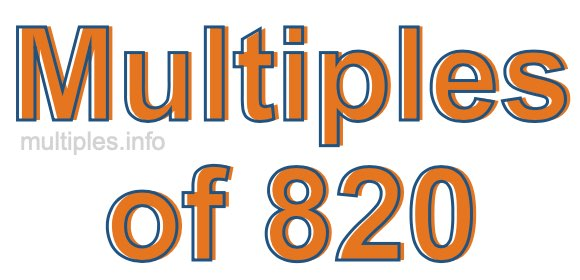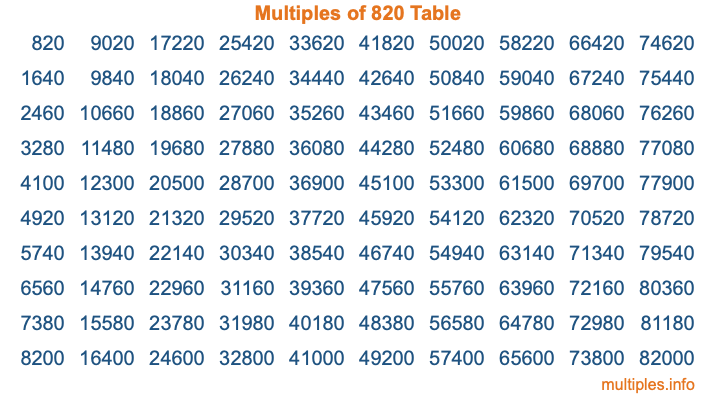Multiples of 820Welcome to the Multiples of 820 page. Here we will first teach you everything you will ever need to know about the multiples of 820, and then give you a study guide summary of everything we taught you to make sure you remember it all. Use this page to look up facts and learn information about the multiples of 820. This page will make you a multiples of eight hundred twenty expert!

Definition of Multiples of 820
Multiples of 820 are all the numbers that when divided by 820 equal an integer. Each of the multiples of 820 are called a multiple. A multiple of 820 is created by multiplying 820 by an integer.

Therefore, to create a list of multiples of 820, you start with 1 multiplied by 820, then 2 multiplied by 820, then 3 multiplied by 820, and so on for as long as you want. Thus, the list of the first five multiples of 820 is 820, 1640, 2460, 3280, and 4100. To see a larger list of multiples of 820, see the printable image of Multiples of 820 further down on this page. We also have a category where you can choose any nth multiple of 820.

Multiples of 820 Checker
The Multiples of 820 Checker below checks to see if any number of your choice is a multiple of 820. In other words, it checks to see if there is any number (integer) that when multiplied by 820 will equal your number. To do that, we divide your number by 820. If the the quotient is an integer, then your number is a multiple of 820.

Is  a multiple of 820?

Least Common Multiple of 820 and ...
A Least Common Multiple (LCM) is the lowest multiple that two or more numbers have in common. This is also called the smallest common multiple or lowest common multiple and is useful to know when you are adding our subtracting fractions. Enter one or more numbers below (820 is already entered) to find the LCM.

Check out our LCM Calculator if you need more details about the Least Common Multiple or if you need the LCM for different numbers for adding and subtraction fractions.

nth Multiple of 820
As we stated above, 820 is the first multiple of 820, 1640 is the second multiple of 820, 2460 is the third multiple of 820, and so on. Enter a number below to find the nth multiple of 820.

th multiple of 820

Multiples of 820 vs Factors of 820
820 is a multiple of 820 and a factor of 820, but that is where the similarities end. All postive multiples of 820 are 820 or greater than 820. All positive factors of 820 are 820 or less than 820.

Below is the beginning list of multiples of 820 and the factors of 820 so you can compare:

Multiples of 820: 820, 1640, 2460, 3280, 4100, etc.

Factors of 820: 1, 2, 4, 5, 10, 20, 41, 82, 164, 205, 410, 820

As you can see, the multiples of 820 are all the numbers that you can divide by 820 to get a whole number. The factors of 820, on the other hand, are all the whole numbers that you can multiply by another whole number to get 820.

It's also interesting to note that if a number (x) is a factor of 820, then 820 will also be a multiple of that number (x).

Multiples of 820 vs Divisors of 820
The divisors of 820 are all the integers that 820 can be divided by evenly. Below is a list of the divisors of 820.

Divisors of 820: 1, 2, 4, 5, 10, 20, 41, 82, 164, 205, 410, 820

The interesting thing to note here is that if you take any multiple of 820 and divide it by a divisor of 820, you will see that the quotient is an integer.

Multiples of 820 Table
Below is an image of the first 100 multiples of 820 in a table. The table is in chronological order, column by column. The first column has the first ten multiples of 820, the second column has the next ten multiples of 820, and so on.The Multiples of 820 Table is also referred to as the 820 Times Table or Times Table of 820. You are welcome to print out our table for your studies.

Negative Multiples of 820
Although not often discussed or needed in math, it is worth mentioning that you can make a list of negative multiples of 820 by multiplying 820 by -1, then by -2, then by -3, and so on, to get the following list of negative multiples of 820:

-820, -1640, -2460, -3280, -4100, etc.

Multiples of 820 Summary
Below is a summary of important Multiples of 820 facts that we have discussed on this page. To retain the knowledge on this page, we recommend that you read through the summary and explain to yourself or a study partner why they hold true.

There are an infinite number of multiples of 820.

A multiple of 820 divided by 820 will equal a whole number.

820 divided by a factor of 820 equals a divisor of 820.

The nth multiple of 820 is n times 820.

The largest factor of 820 is equal to the first positive multiple of 820.

820 is a multiple of every factor of 820.

820 is a multiple of 820.

A multiple of 820 divided by a divisor of 820 equals an integer.

820 divided by a divisor of 820 equals a factor of 820.

Any integer times 820 will equal a multiple of 820.

Multiples of a Number
Here you can get the multiples of another number, all with the same attention to detail as we did for multiples of 820 on this page.

Multiples of
Multiples of 821
Did you find our page about multiples of eight hundred twenty educational? Do you want more knowledge? Check out the multiples of the next number on our list!